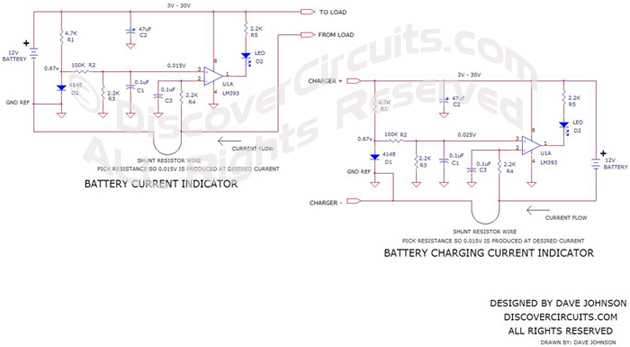DiscoverCircuits.com, has 45,000+ electronic circuits, cross-referenced into 500+ categories. We have searched the web to help you find quick solutions & design ideas.
 HOME Schematics Index Dave's Hobby Corner Dave's Circuits Contact Info
 Imagineering Ezine Dave Johnson, P.E. Faraday Touch Switches
 Got Designs? Please eMail if you want me to link to and/or post your original design.  NOTE:  We make every effort to link to original material posted by the designer.  Please contact us if our link is not to your site!  Thanks.DiscoverCircuits.com -- Hobby Corner Last Updated on: Wednesday, June 02, 2021 01:17 PM Hobby Circuits List The contents & graphics of Discovercircuits.com are copyright protected. LINK to Dave's circuit, but DO NOT COPY any files to your WEB SITE server

DC CURRENT INDICATOR #4    December 15, 2008

The circuit below uses some common components to turn on an LED whenever DC current above a certain level is detected.  The circuit uses a very popular LM393 dual voltage comparator from National Semiconductor and a common 1N4148 signal diode.  The diode acts as a crude 0.7v voltage reference.  Only one of the comparators inside the 8 pin package is used.  A pair of resistors across the diode forms a voltage divider, which produce a reference voltage of about 0.015v. The circuit compares this reference voltage to a voltage produced across a current shunt resistor.  A section of wire already in place can be used as the current shunt.  The circuit can be used to indicate current drawn from a battery and routed to a load or current from a battery charger to the battery. Both circuits are shown on the drawing.  The circuit shows components for a 12v system but it will work well using any DC supply from 3v to 30v.

The circuit is sensitive enough that it can indicate fairly small currents through a section of thick wire.   As an example, an 18ga wire only 24 inches long will have a resistance of about 0.013 ohms.  The needed 0.015 volts will be generated with a current flow of about 1.2 Amps. If more sensitivity is needed, a longer section of wire can be used as a shunt resistor.

Use Ohm’s Law to calculate the resistance needed.  Ohm’s Law equation is: E = RI, where R is the resistance in ohms, E is the voltage developed across the resistance in volts and I is the current flowing through the resistor in Amps.  If we set E at 0.015 volts and R at 0.0012 ohms, then I = 1.2 Amps.

Here is one example how this circuit might be used.  Suppose you have a large 12v battery, which supplies current to various loads inside a recreational vehicle (RV).  You noticed that a 10ga wire 10 feet long connects the battery negative terminal to a junction box.  You look up the resistance of the 10ga wire from a chart and find out that the typical resistance is about 0.001 ohms per foot.  Therefore, if you used the battery negative terminal as one point of reference and the connection in the junction box as another point, then the resistance should be about 0.01 ohms.  If the current indicator circuit were connected between those two points, then the circuit should switch on the LED whenever current exceeding 1.5 Amps is drawn from the battery.
In another example, suppose you have a battery changer connected to a 12v lead acid battery.  The charger uses a pair of 18ga wires 12 feet long.  The resistance of 18ga wire is about 0.0064 ohms per foot, so the resistance of one of the 12 foot wires should be about 0.077 ohms.  Using the current indicator circuit and using the two ends of the cable as reference points, the LED should turn on whenever the charging current exceeds 0.20 Amps.  The indicator light should turn off when the battery is fully charged.

Here  is a link to a Table for Copper Wire

Click on Drawing Below to view PDF version of SchematicHOME Schematics Index Hobby Corner Dave's Circuits Contact Info
 Imagineering Ezine Dave Johnson, P.E. Faraday Touch Switches